Electron. J. Diff. Eqns., Vol. 2004(2004), No. 72, pp. 1-25.

### Exact multiplicity results for a p-Laplacian positone problem with concave-convex-concave nonlinearities Idris Addou & Shin-Hwa Wang

Abstract:
We study the exact number of positive solutions of a two-point Dirichlet boundary-value problem involving the p-Laplacian operator. We consider the case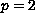and the case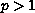, when the nonlinearity satisfies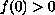(positone) and has three distinct simple positive zeros and such that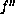changes sign exactly twice on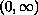. Note that we may allowto change sign more than twice on. We also present some interesting examples.

Submitted March 8, 2004. Published May 20, 2004.
Math Subject Classifications: 34B18, 34B15.
Key Words: Exact multiplicity result, p-Laplacian, positone problem, bifurcation, concave-convex-concave nonlinearity, positive solution, dead core solution, time map.

Show me the PDF file (1398K), TEX file, and other files for this article.Idris Addou Departement de Mathematiques et Statistiques Universite de Montreal C.P. 6128, Succ. Centre-ville, Montreal, Quebec, Canada, H3C2J7 email: addou@dms.umontreal.ca Shin-Hwa Wang Department of Mathematics National Tsing Hua University Hsinchu, Taiwan 300, Republic of China email: shwang@math.nthu.edu.tw